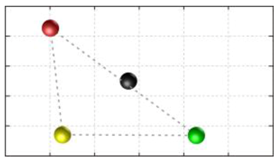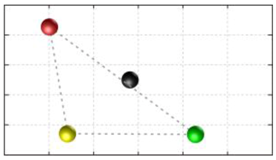Next Article in Journal
A Survey of Wall Climbing Robots: Recent Advances and Challenges
Open AccessArticle

# Trajectory Generation and Stability Analysis for Reconfigurable Klann Mechanism Based Walking Robot

byJaichandar Kulandaidaasan Sheba 1,2,*,Mohan Rajesh Elara 3,Edgar Martínez-García 2 andLe Tan-Phuc 1
1
School of Electrical and Electronics Engineering, Singapore Polytechnic, Singapore 6751133, Singapore
2
Institute of Engineering and Technology, Universidad Autónoma de Ciudad Juárez, Cd Juárez 32310, Mexico
3
Engineering Product Development Pillar, Singapore University of Technology and Design, Singapore 487372, Singapore
*
Author to whom correspondence should be addressed.
Robotics 2016, 5(3), 13; https://doi.org/10.3390/robotics5030013
Received: 24 March 2016 / Revised: 22 June 2016 / Accepted: 22 June 2016 / Published: 30 June 2016

## Abstract

Reconfigurable legged robots based on one degree of freedom are highly desired because they are effective on rough and irregular terrains and they provide mobility in such terrain with simple control schemes. It is necessary that reconfigurable legged robots should maintain stability during rest and motion, with a minimum number of legs while maintaining their full range of walking patterns resulting from different gait configuration. In this paper we present a method to generate input trajectory for reconfigurable quadruped robots based on Klann mechanism to properly synchronize movement. Six useful gait cycles based on this reconfigurable Klann mechanism for quadruped robots has been clearly shown here. The platform stability for these six useful gait cycles are validated through simulated results which clearly shows the capabilities of reconfigurable design.

## 1. Introduction

Legged robots are widely used for a variety of applications, especially in the area of search and rescue operations for their ability to handle uneven terrain . Legged robots are designed and programmed to respond to various needs based on the task, and offer better stability, manoeuvrability, and energy efficiency. However, any changes in the gait configuration based on the task leads to numerous opportunities as well as research challenges. Legged animals, depending on the task or behaviour, can coordinate a wide range of components and systems to adapt effectively under various conditions such as running, walking, chasing, courtship, and attack [2,3].
Various design strategies to generate different gait patterns in legged robots are found in robotic literature. For legged robots, there are many different approaches to generate gait pattern, including: genetic-fuzzy system , reinforcement learning , evolutionary approach , graph search approach , mechanical energy constraints , or neural networks [9,10]. Using one degree of freedom based on a reconfiguration planar mechanism, simple control schemes can be proposed as an alternative method . In [12,13], the authors present the Klann based reconfigurable design and implementation where a robot changes its structural morphology by changing its components and sub-assembly parameters to adapt to multi-terrain and multi-tasking by producing a wide set of novel gait patterns.
The fundamental challenge in reconfigurable legged robots and associated gait generation is to reach stability in both motion and rest with the minimum number of legs, with a complete range of walking patterns. For quadruped robots with one degree of freedom and reconfigurable legs, input trajectories to synchronize different gait cycles has been discussed in detail in this paper. To clearly explain our approach, we focused on a four legged robot with reconfigurable Klann legs (Figure 1). By reconfiguring the Klann based legs, six theoretically useful walking patterns resulting from different gait cycles have been achieved. In this paper it is shown that by using the proposed technique, useful feasible patterns can be successfully obtained. By applying the following method, the capabilities of standard non-reconfigurable quadruped Klann legs are significantly extended.
The rest of this paper is organized as follow: Section 2 presents the specifications of the reconfigurable Klann platform under testing. Section 3 deals with the design of Trajectory Generation. Section 4 explains the synchronization method used to coordinate multiple legs to achieve the six distinct  stable walking patterns. Section 5 presents the static stability analysis of the realized gait. Section 6 deals with the static and in motion characterization of transformation. Section 7 deals with the static stability analysis of the transformation. Section 8 gives a conclusion of this study along with a discussion regarding future work.

## 2. Specification of the Reconfigurable Klann Platform

In this section, specifications of a developed reconfigurable Klann legged robot is discussed. This designed reconfigurable Klann based walking platform is used herein as a case study for the generation of input joint trajectories to synchronize multiple reconfigurable one-degree-of-freedom legs for realizing stable walking gaits. In our previous work, we have presented a novel approach that produces a wide range of usable gait curves for Klann based reconfigurable legged robots during locomotion . By changing its link length parameter, such a robot can vary its hardware morphology. During the reconfiguration process the recommended mechanism switches from a pin jointed Gürbler kinematic chain to a seven degree-of-freedom mechanism with slider joints. The proposed design, while maintaining efficiency, extends the capability of the original design to not only produce distinctive useful gait patterns but realize behaviour beyond locomotion. The identified novel gait patterns are shown in Table 1.
For most legged platforms, one of the important factors is to determine the required number of legs to obtain stability during motion and rest. Previous works related to legged platforms utilize more than four legs to overcome stability issues . The challenge faced with the legged platform is that increase in the number of legs to overcome stability issues will give rise to other problems such as cost, size, complex control system, and associated maintenance issues. For a platform with reconfigurable legs, this problem is even more obvious because of changing leg dimensions associated with the task. Thus, increasing the number of legs will directly contribute to the complexity of the entire system. As discussed, it is important to develop a reconfigurable walking platform with a minimum number of legs. However, reconfigurable designs with less than four legs should be avoided in order to maintain simplicity and at the same time to avoid the related considerations of Zero Moment Point (ZMP) approaches for achieving static and/or dynamic stability [15,16,17,18]. In addition, toe slipping due to difference in leg speed during realization of a walking pattern need to be taken into consideration . As a consequence, a four-legged reconfigurable Klann platform is considered for the analysis discussed in this work. Figure 1 shows the CAD design of the four-legged Reconfigurable Klann platform. Using this robot, with reconfigurable capabilities, the results of six gait patterns are tabulated in Table 1. Each leg is controlled independently by a rotary actuator with its control system. In the following section, the formulation for the trajectory generation of input joints is presented.

## 3. Trajectory Generator Planning

In this paper, we use cubic polynomial interpolation to help the leg generate a smooth trajectory from starting point to ending point. The input angle and speed are represented as third and second degree. Since the position and velocity of the crank are controlled through a continuous function with a degree higher than one, the load force applied to the main actuator can be decreased to a minimum. Because of these advantages, we have used this method for our trajectory generation. This approach has already been successfully used in the community [19,20,21].
We define the input angle (crank) as $r ( t )$ and the speed of the crank as $r ˙ ( t )$ in term of time $t$. The cubic polynomial interpolation function can be defined as follow:
$r ( t ) = a 3 t 3 + a 2 t 2 + a 1 t + a 0$
$r ˙ ( t ) = 3 a 3 t 2 + 2 a 2 t + a 1$
where represents coefficients and these are derived from two consecutive points in a set of four ($θ A$, $θ B$, $θ C$, $θ D$) of a given trajectory. The first point can be considered as the initial state ($θ s$) and the next point as the final state ($θ f$) where the execution time is from 0 to $T$ (constant) (s). From the initial state, $a 0$ and $a 1$ can be derived:
$r ( 0 ) = a 0 = θ s$
$r ˙ ( 0 ) = a 1 = θ ˙ s$
With the final state (which is already planned), we have:
$r ( T ) = a 3 T 3 + a 2 T 2 + a 1 T + a 0 = θ f$
$r ˙ ( T ) = 3 a 3 T 2 + 2 a 2 T + a 1 = θ ˙ f$
From Equations (3)–(6) we have:
${ a 3 T 3 + a 2 T 2 = θ f − θ s − θ ˙ s T 3 a 3 T 2 + 2 a 2 T = θ ˙ f − θ ˙ s ⇨ { a 2 = 3 θ f − 3 θ s − θ ˙ f T − 2 θ ˙ s T T 2 a 3 = − 2 θ f + 2 θ s + θ ˙ f T + θ ˙ s T T 3$
Then, from Equations (3), (4) and (7) we have all the coefficients .
Using the Trajectory Generation method as earlier explained, our strategy for synchronization is designed. As the third degree polynomial interpolation can be calculated uniquely, based upon the initial condition $θ s , θ ˙ s$, the final conditions $θ f , θ ˙ f$, and the transformation time $T$, the synchronization strategy is therefore designed by defining these states.

## 4. Walking Phase Synchronization

Apart from planning a suitable trajectory corresponding to different terrains, reconfigurable quadrupled Klann platforms must have a leg synchronization strategy to achieve a stable walking gait. Several locomotion patterns including “walk”, “trot”, “bound”, and “gallop” as in Figure 2 are available from the literature  for the case of a general multi-legged robot. Specifically, in this part, we focus on the synchronization strategy for the “walk” pattern. The other three patterns, where only two legs contact the ground surface at a specific time, are left for future work. Figure 2 (left) shows the overall definition for all four legs of the robot as well as viewing directions that will be used to evaluate the input angles of the four legs in Table 1.
It can be seen from Figure 2 that in a walk pattern, there is a shift of 90 degrees among the four legs’ phases. “Transfer” and “support” are used as terms for describing states of the leg that swings over the ground and touches the ground respectively. At any moment, only one leg is in transfer phase while the other three legs are in support phase to carry the mass of robot. The switching sequence of the transfer leg is “Left-Fore” → “Right-Hind” → “Right-Fore” → “Left-Hind”, and so on. The trajectory generator using cubic polynomial interpolation introduced in Section 3 is used to produce a smooth transition from current state to next one. Since Klann is a one degree-of-freedom linkage, the end point of the leg depends only on the angle of the driving link, the input angle, defined as $θ$, and the support leg and the transfer leg can be switched depending on the value of the angle. For the purpose of defining the order of the states through naming convention, we define the states of the transfer leg “Left-Fore”, “Right-Hind”, “Right-Fore”, and “Left-Hind” as “Phase 1”, “Phase 2”, “Phase 3”, and “Phase 4”, respectively. The switch angles of the support and transfer legs for gaits are presented in Table 2 and Figure 3 where the support state starts from angle $θ B$ and ends at $θ A$ to switch to transfer state. Figure 4 shows the coordinate systems used to analyse input angle for the four legs. Figure 5 shows the relationship between input angles and $θ D$ and the position of the leg for standard curve trajectory. The other two states, $θ C$ and $θ D$, are calculated as follows:
$η = θ A − θ B 3$
$θ C = θ B + η$
$θ D = θ B + 2 η$
For the “Standard curve”, $θ A$ and $θ B$ are defined by the input angle at points on the curve that have the same height with the uppermost point at the bottom of the curve. For the “Digitigrade locomotion” and “Hammering motion”, due to short contact to the ground, $θ A$ and $θ B$ are chosen by the angle of curvature at 20% of the total height of trajectory from the lowest point of the gait pattern.
In the case of “Digging motion”, $θ B$ is defined by the angle of curvature at the lowest vertical height point of the gait pattern and $θ A$ is defined by the angle of curvature of the middle point on the left of the gait pattern. For “Jam avoidance”, the start of the support phase is chosen at the rightmost point on the curve with corresponding input angle $θ B$ while the end of this phase is placed at the leftmost point with angle $θ A$. And finally, for “Step climbing”, $θ B$ and $θ A$ are determined by the time the leg starts to climb on step and release of the leg from the step respectively as shown in Figure 3.
The initial states of each leg are presented in Table 3 (column “Phase 1”) and the final states (Phase 4) of each leg are the initial states of the next cycle. Figure 6 shows the four leg walking synchronization for all the four phases. The position of each leg is clearly shown for the standard Klann curve. Figure 7 shows all possible states of stable walking of a Klann standard trajectory with half-phase shift. By using cubic polynomial interpolation for all trajectories generated that are shown in Figure 8, it can be seen that the transition of the input angle between phases over time is very smooth and without any sudden change, which helps to avoid impact on the actuators and linkages.

## 5. Static Stability Analysis of Gaits

In this section, we discuss the static stability of walking for the quadruped robot. The quadruped robot walks with three support leg and one transfer leg. If the center of gravity (COG) stays inside the supporting leg polygon, the robot is stable, and in the case of the quadruped robot, its supporting leg polygon is a triangle. In addition, four kinds of triangles can exist as shown in Figure 9. From Figure 9, it is confirmed that all supporting leg polygons consist of two legs in opposing corner and one other leg. Whenever the COG of the robot is placed on its center, the positional relationship between a line on the two legs in opposing corners and the COG has an influence on its static stability. Figure 10 shows two situations of static stability, stable and unstable condition.
Next, we will evaluate the static stability of the whole platform at a specific moment of each phase corresponding to the proposed gaits. The relationship between the center of gravity (COG) and the three support legs of the robot is presented in Table 4 where the black points denote the COG of the robot, the yellow points, green points, blue points and red points represent the contact points of the support legs with the ground, and the gray line shows the static stability zone. All the COG results are automatically calculated and projected using the software Autodesk Inventor. These static stability results show that in most cases, the proposed approach generates sufficient conditions to keep the platform stable. This is observed by the position of the center of gravity inside the static stability zone.
Although the entire COG results are inside the static stability zone, low stability margins are witnessed in Table 4 when computed by known evaluation methods [23,24,25]. For such narrow stability margins, there are several solutions to improve stability while still maintaining simplicity of control. One example is to add an inverted pendulum to act as a counterweight that moves oppositely to the transfer leg direction. In any case, this subject warrants further research.

## 6. Characterization of Transformation

In the previous sections, we have shown stable walking by the Klann reconfigurable platform using phase synchronization, trajectory generation, as well as analysis of the static stability. The next challenge is to develop a stable transformation. Some transformation methods for the multi-legged robot are known, such as transforming on the spot  or transforming during walking . In this paper, we implement both these transformation methods on our reconfigurable Klann robot. The first method (“Static Transformation” in this paper) will transform all four legs of the robot at the same time while the robot is in rest state. This will be an advantage for the robot when transforming in a small space as well as keeping the robot stable because no leg swings over the ground. For the second method (“Transformation in Walking” in this paper), the robot transforms leg by leg in its transfer phase while walking in order to save time and perform a high maneuverability. Cubic polynomial interpolation is also applied to both methods to provide a smooth transformation by substituting the initial and final state into the trajectory generator in the same way as Section 4. For this case, not only the input angle of the driving link but also the link dimensions have to be calculated through trajectory generation Equation (1). Let us define shown in Figure 11, as the angle of the driving link of leg $i$, as the length of the changing links ( for “Jam avoidance” to “Digging motion” in this paper) of leg $i$, and $Δ y i$ as the offset height of each leg after transformation. Moreover, and $y ′$ represent those parameters after transformation.

#### 6.1. Transformation I—Static Transformation

In this method, the robot performs a transformation in resting state, where all four legs transform at the same time and the whole platform can change the vertical height due to the characteristics of different gaits. In addition, the robot transforms under the state that all legs contact the ground to improve the stability with the following procedure:
• Stop all legs at their initial state of gait (column “Phase 1” in Table 3).
• Perform a transformation for both input driving angle and link dimension.
• Start to walk again.
Figure 12a–d shows the transformation from Jam avoidance to Digging motion as an example following several steps presented in  without the high compensation step. Trajectory generation is implemented for the input angle changing process from $θ i$ to $θ i ′$ and link dimension $l i$ to $l i ′$ with $i = 1 , … , 4$ shown in Figure 12e,f.

#### 6.2. Transformation II—Transformation in Motion

In this method, basically we combine walking phase synchronization together with transformation leg by leg. The transformation is applied when the leg swings over the ground to switch from support to transfer phase using trajectory generation designed in Section 4 and Table 3. Since the gait pattern before and after transformation is mixed, height difference between the four support legs will become large. In such cases, the linear height compensator is used to prevent undesired floor contact of transformed leg.
In our previous study , the height of the whole leg was decided by the angle of the driving link and the link dimensions since the leg transformed between the lowest points of each gait pattern. On the other hand, in this study, the height offset for the transforming leg is also applied by the designed trajectory generator. The trajectory of the whole transformation process from Jam avoidance to digging motion is shown in Figure 13k. Before transforming, the leg moves with Jam avoidance trajectory until it reaches point “A”, also known as the start point of the transfer phase of Jam avoidance. Then, the transformation process is started with changes in the input angle from $θ A$ of Jam to $θ B$ of Digging as well as the transforming links dimensions and height offset. During transformation, the trajectory of the leg is illustrated as the blue line in Figure 13k. The process ends at point “B”, which is also the beginning of Phase 2 of Digging motion, to generate a new trajectory. This process is repeated for the other three legs with the sequence mentioned in Section 4 and Table 3.
Notice the pink line connecting the Left-Fore leg’s frame with the body platform in the red circle in Figure 13 and Figure 14. It represents height offsetting of the linear slider to prevent undesired floor contact during transformation ($Δ y$). The length of this line depends on which transformation is performed between two patterns in a set mentioned in Table 1 (Standard curve, Jam avoidance, digitigrade motion, Digging motion, and Hammering motion).

## 7. Static Stability Analysis of the Transformation

The static stabilities for two types of transformation are evaluated. We first discuss the stabilities of the static transformation. The main point of static transformation stability is to transform from the initial state of all four legs shown in Figure 3 and Table 3. Since the robot transforms under the state that all legs are contacting with the ground, the robot can keep its static stability continuously during transformation. In this case, height difference between the leg before and after transformation will be the same for all four legs, therefore, undesired floor contact is eliminated and height offset is not necessary.
Next, stabilities of the transformation in walking are reviewed. Relationships between the support legs and the COG of the platform for all available transformations are automatically calculated and projected from top to bottom using the Dynamic Simulation tool in Autodesk Inventor in an ideal environment, without any external force application on the robot and all parts made from the same material with the same characteristics.
Phase 1 and Phase 4 of this transformation method has similar stability as shown in Table 4 because all support legs are in the same pattern. Hence, stability analysis is needed only for Phase 2 and Phase 3. Here, only static stability analysis is considered for Phase 2. The initial and final state of the transfer leg in the transformation in walking is shown in Table 5. In Table 6, black points represent the COG of the platform, and yellow points, blue points, green points, and red points represent the contacting point of the support legs. The gray lines show the static stability zone. From Table 6, it can be assumed that the transformation in walking is stable by the proposed method because the center of gravity stays inside the static stability zone, except for some situations marked with (*).

## 8. Conclusions

A novel design approach towards the development of a trajectory generator to realize a set of stable walking patterns for four legged Klann based reconfigurable robots has been presented in this paper. Leg synchronization challenges in reconfigurable design and novel approaches to address them have been presented here supported by simulation studies. To validate the proposed method, stability analysis of six prospective gait patterns have been discussed here. Robots that can adapt to situations and generate appropriate stable gaits would extend their capabilities beyond intended applications. Currently a four legged reconfigurable robot based on the Klann mechanism is being constructed to validate the robustness of the produced gaits and control approaches. Future work would include online gait generation and transformation via the use of gait libraries in relation to the terrain under testing. A number of precedents [26,27,28] exists in literature to this end, and by using these works as a base, we could extend the locomotion capabilities of our reconfigurable Klann platform. Another potential design optimization problem considered for future work is to minimize the number of active actuators used for locomotion from four to two in the current mechanical design.

## Author Contributions

Jaichandar Kulandaidaasan Sheba: conceived and designed the experiments, wrote the paper Mohan Rajesh Elara: Mentoring, Reviewing, analyzed the data. Martinez-Garcia Edgar, Mentoring, Reviewing, analyzed the data. Le Tan-Phuc: Hardware and software design, Experiment validation.

## Conflicts of Interest

The authors declare no conflict of interest.

## References

1. Kajita, S.; Espiau, B. Legged robots. In Handbook of Robotics; Siciliano, B., Khatib, O., Eds.; Springer: Berlin/Heidelberg, Germany, 2008. [Google Scholar]
2. Chen, X.; Wang, L.; Ye, X.; Wang, G.; Wang, H. Prototype development and gait planning of biologically inspired multi-legged crablike robot. Mechatronics 2013, 23, 429–444. [Google Scholar] [CrossRef]
3. Ghassaei, A. The Design and Optimization of a Crank-Based Leg Mechanism. Ph.D. Thesis, Pomona College, Pomona, CA, USA, 2011. [Google Scholar]
4. Jha, R.K.; Singh, B.; Pratihar, D.K. Online stable gait generation of a two-legged robot using a genetic–fuzzy system. Robot. Auton. Syst. 2005, 53, 15–35. [Google Scholar] [CrossRef]
5. Erden, M.S.; Leblebicioğlu, K. Free gait generation with reinforcement learning for a six-legged robot. Robot. Auton. Syst. 2008, 56, 199–212. [Google Scholar] [CrossRef]
6. Chernova, S.; Veloso, M. An evolutionary approach to gait learning for four-legged robots. In Proceedings of the 2004 IEEE/RSJ International Conference on Intelligent Robots and Systems (IROS 2004), Sendai, Japan, 28 September–2 October 2004; Volume 3, pp. 2562–2567.
7. Pal, P.K.; Jayarajan, K. Generation of free gait-a graph search approach. IEEE Trans. Robot. Autom. 1991, 7, 299–305. [Google Scholar] [CrossRef]
8. Asano, F.; Yamakita, M.; Kamamichi, N.; Luo, Z.W. A novel gait generation for biped walking robots based on mechanical energy constraint. IEEE Trans. Robot. Autom. 2004, 20, 565–573. [Google Scholar] [CrossRef]
9. Chiel, H.J.; Beer, R.D.; Quinn, R.D.; Espenschied, K.S. Robustness of a distributed neural network controller for locomotion in a hexapod robot. IEEE Trans. Robot. Autom. 1992, 8, 293–303. [Google Scholar] [CrossRef]
10. Arena, P.; Fortuna, L.; Frasca, M.; Patane, L. Learning anticipation via spiking networks: Application to navigation control. IEEE Trans. Neural Netw. 2009, 20, 202–216. [Google Scholar] [CrossRef] [PubMed]
11. Nansai, S.; Rojas, N.; Elara, M.R.; Sosa, R. Exploration of adaptive gait patterns with a reconfigurable linkage mechanism. In Proceedings of the IEEE/RSJ International Conference on Intelligent Robots and Systems (IROS), Tokyo, Japan, 3–7 November 2013; pp. 4661–4668.
12. Klann, J.C. Walking Device. Google Patents U.S. 6,478,314 B1, 12 November 2002. [Google Scholar]
13. Sheba, J.K.; Mohan, R.E.; Martínez-García, E.; Tan-Phuc, L. Synthesizing reconfigurable foot traces using a Klann mechanism. Robotica 2015, 1–17. [Google Scholar] [CrossRef]
14. Komoda, K.; Wagatsuma, H. A study of availability and extensibility of Theo Jansen mechanism toward climbing over bumps. In Proceedings of the 21st Annual Conference of the Japanese Neural Network Society, Okinawa, Japan, 15–17 December 2011.
15. Erbatur, K.; Okazaki, A.; Obiya, K.; Takahashi, T.; Kawamura, A. A study on the zero moment point measurement for biped walking robots. In Proceedings of the 7th International Workshop on Advanced Motion Control, Maribor, Slovenia, 3–5 July 2002; pp. 431–436.
16. Kajita, S.; Kanehiro, F.; Kaneko, K.; Fujiwara, K.; Harada, K.; Yokoi, K.; Hirukawa, H. Biped walking pattern generation by using preview control of zero-moment point. In Proceedings of the 2003 IEEE International Conference on Robotics and Automation, Taipei, Taiwan, 14–19 September 2003; pp. 1620–1626.
17. Sardain, P.; Bessonnet, G. Forces acting on a biped robot center of pressure—Zero moment point. IEEE Trans. Syst. Man Cybern. 2004, 34, 630–637. [Google Scholar] [CrossRef]
18. Nansai, S.; Mohan, R.; Iwase, M. Dynamic analysis and modeling of Jansen mechanism. Procedia Eng. 2013, 64, 1562–1571. [Google Scholar] [CrossRef]
19. De Britto Vidal Filho, W.; Bezerra, J.M. Mechatronic design of a chair for disabled with locomotion by legs. ABCM Symp. Ser. Mechatron. 2012, 5, 1142. [Google Scholar]
20. Rojas, N.; Thomas, F. On closed-form solutions to the position analysis of Baranov trusses. Mech. Mach. Theory 2012, 50, 179–196. [Google Scholar] [CrossRef][Green Version]
21. Rojas, N. Distance-based formulations for the position analysis of kinematic chains. Ph.D. Thesis, Institut de Robòtica i Informàtica Industrial, Barcelona, Spain, 2012. [Google Scholar]
22. Muybridge, E. Animals in Motion; Dover Publications, INC.: New York, NY, USA, 1957. [Google Scholar]
23. Yoneda, K.; Hirose, S. Tumble stability criterion of a walking machine. J. Robot. Soc. Jpn. 1996, 14, 517–522. (In Japanese) [Google Scholar] [CrossRef]
24. Hirise, S.; Tsukakoshi, H.; Yoneda, K. Static stability criterion for walking robots on irregular terraings. J. Robot. Soc. Jpn. 1998, 16, 1076–1082. (In Japanese) [Google Scholar] [CrossRef]
25. Messuri, D.A.; Klein, C.A. Automatic body regulation for maintaining stability of a legged vehicle during rough-terrain locomotion. IEEE J. Robot. Autom. 1985, 1, 132–141. [Google Scholar] [CrossRef]
26. Mark, M.; Sucan, I.A.; Kavraki, L.E. Benchmarking motion planning algorithms: An extensible infrastructure for analysis and visualization. IEEE Robot. Autom. Mag. 2015, 96–102. [Google Scholar] [CrossRef]
27. Kaltsoukalas, K.; Makris, S.; Chryssolouris, G. On generating the motion of industrial robot manipulators. Robot. Comput. Integr. Manuf. 2015, 32, 65–71. [Google Scholar] [CrossRef]
28. Voss, C.; Moll, M.; Kavraki, L.E. A heuristic approach to finding diverse short paths. In Proceedings of the 2015 IEEE International Conference on Robotics and Automation (ICRA), Seattle, WA, USA, 26–30 May 2015.
Figure 1. A Legged Robot based on one degree of freedom and reconfigurable planar leg mechanism can be designed to operate in a complex environment with simple control schemes. This four legged robot based on reconfigurable Klann legs is capable of generating up to six different gait cycles.
Figure 1. A Legged Robot based on one degree of freedom and reconfigurable planar leg mechanism can be designed to operate in a complex environment with simple control schemes. This four legged robot based on reconfigurable Klann legs is capable of generating up to six different gait cycles.Figure 2. Overall definition for four legs of quadruped robot (Left) and phase relationship of some locomotion patterns (Right).
Figure 2. Overall definition for four legs of quadruped robot (Left) and phase relationship of some locomotion patterns (Right).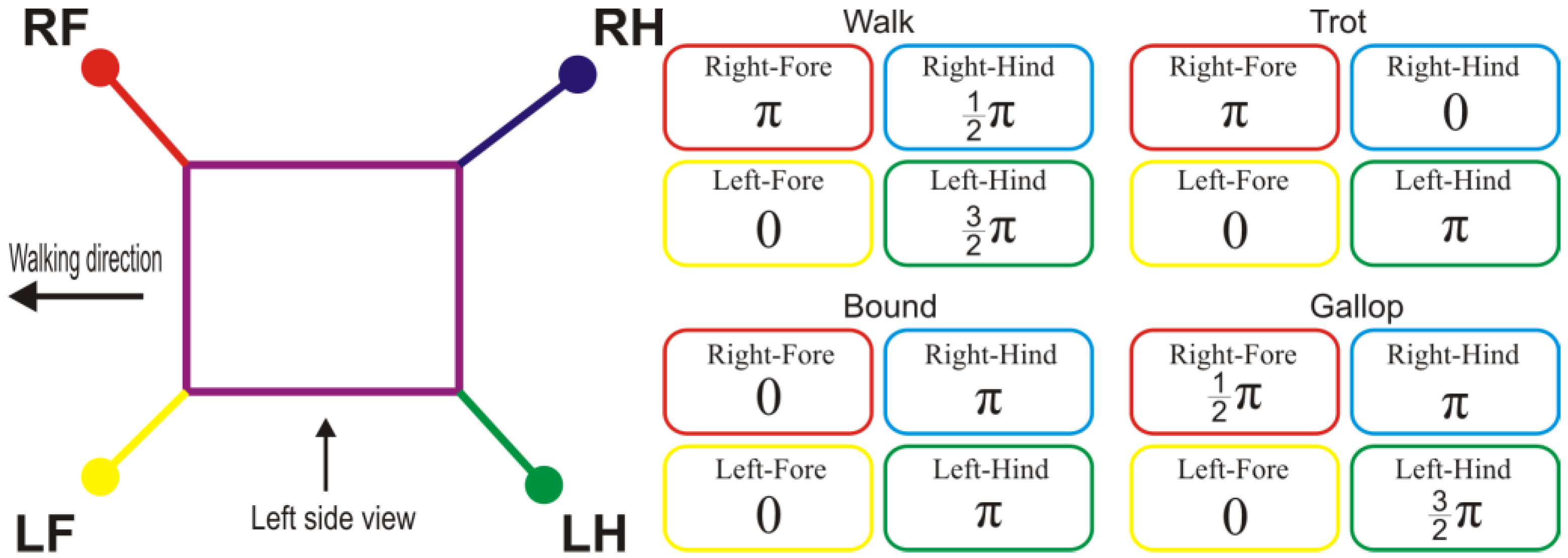Figure 3. The switching input angles between support and transfer phases. The green and red curves represent the leg in transfer and support phase respectively. (a) Standard curve; (b) Jam avoidance; (c) Digitigrade; (d) Step climbing; (e) Digging; (f) Hammering.
Figure 3. The switching input angles between support and transfer phases. The green and red curves represent the leg in transfer and support phase respectively. (a) Standard curve; (b) Jam avoidance; (c) Digitigrade; (d) Step climbing; (e) Digging; (f) Hammering.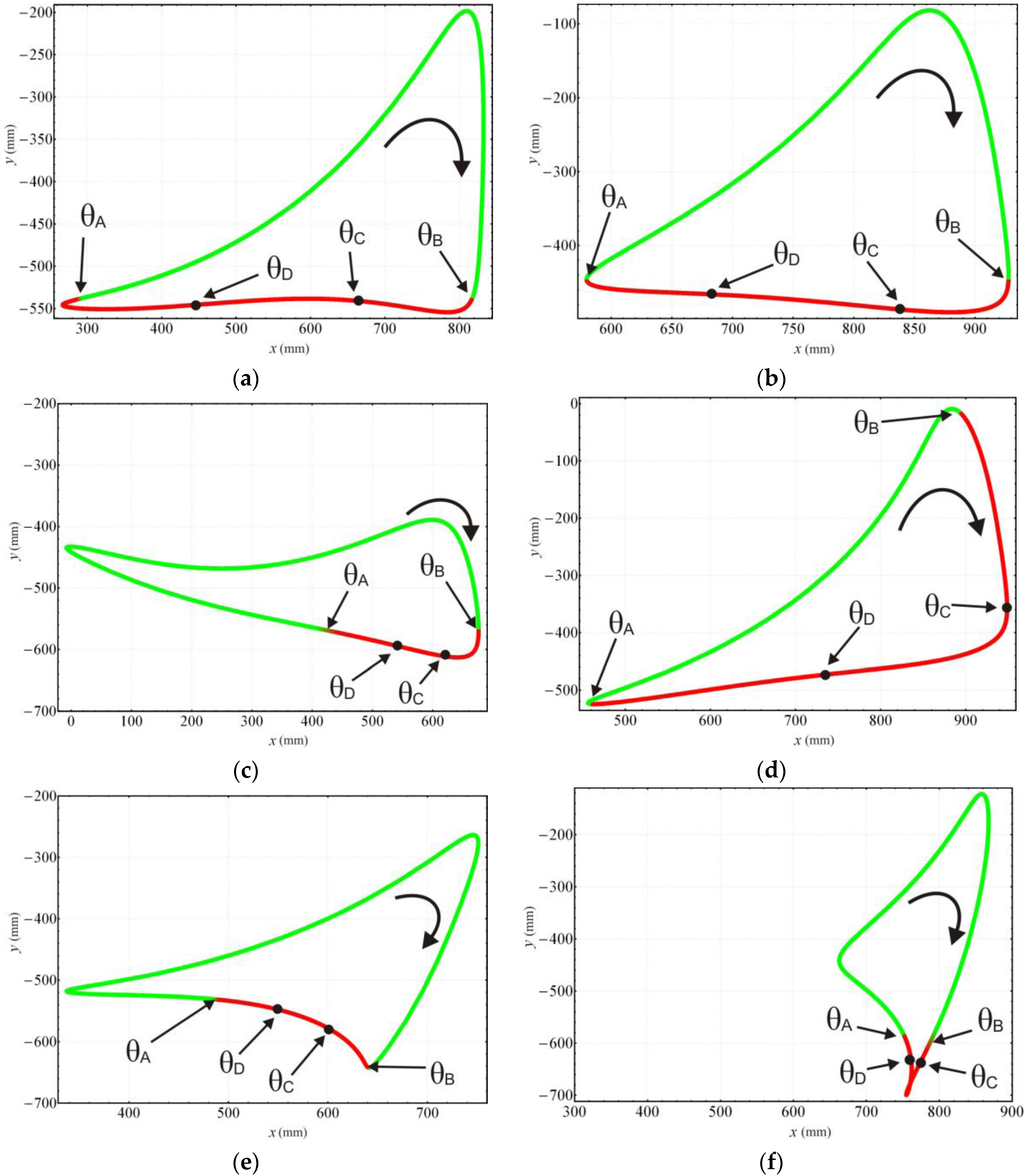Figure 4. Coordinate systems used to analyse input angle for the four legs. represent the centre of the input links of the four legs.
Figure 4. Coordinate systems used to analyse input angle for the four legs. represent the centre of the input links of the four legs.Figure 5. The relationship between input angle ($θ )$ and position of the leg for standard curve trajectory. (a) Phase 1; (b) Phase 2; (c) Phase 3; (d) Phase 4.
Figure 5. The relationship between input angle ($θ )$ and position of the leg for standard curve trajectory. (a) Phase 1; (b) Phase 2; (c) Phase 3; (d) Phase 4.Figure 6. An illustration of four leg walking synchronization for all the four phases given in Table 3. (a) Phase 1; (b) Phase 2; (c) Phase 3; (d) Phase 4.
Figure 6. An illustration of four leg walking synchronization for all the four phases given in Table 3. (a) Phase 1; (b) Phase 2; (c) Phase 3; (d) Phase 4.Figure 7. The simulation result of the phase synchronization of the standard curve for stable walking. The leg is corresponding with the specify color: Yellow-Left Fore leg; Green-Left Hind leg; Red-Right Fore leg; Blue-Right Hind leg. (a) Starting of Phase time = 0; (b) Time = 1 s; (c) Time = 2 s; (d) Time = 3 s; (e) Time = 4 s; (f) Time = 5 s; (g) Time = 6 s; (h) Time = 7 s; (i) Time = 8 s.
Figure 7. The simulation result of the phase synchronization of the standard curve for stable walking. The leg is corresponding with the specify color: Yellow-Left Fore leg; Green-Left Hind leg; Red-Right Fore leg; Blue-Right Hind leg. (a) Starting of Phase time = 0; (b) Time = 1 s; (c) Time = 2 s; (d) Time = 3 s; (e) Time = 4 s; (f) Time = 5 s; (g) Time = 6 s; (h) Time = 7 s; (i) Time = 8 s.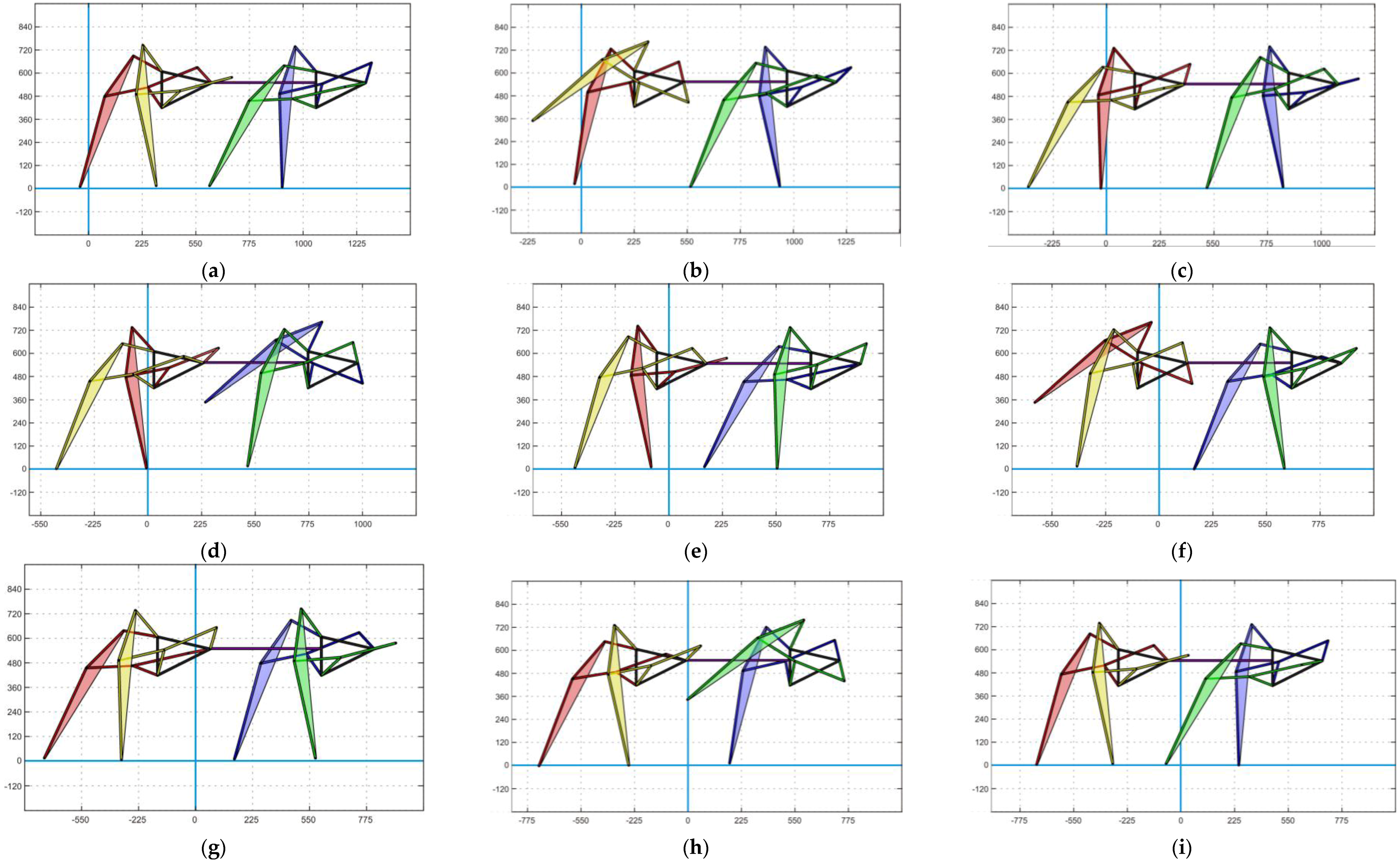Figure 8. Input angle value of four legs in one cycle including four phases, phase transition (Left) and in five cycles of 8 seconds each (Right).
Figure 8. Input angle value of four legs in one cycle including four phases, phase transition (Left) and in five cycles of 8 seconds each (Right).Figure 9. The variety of the supporting leg polygons of the quadruped robot. The grey area represents the static stability zone. (a) Phase 1; (b) Phase 2; (c) Phase 3; (d) Phase 4.
Figure 9. The variety of the supporting leg polygons of the quadruped robot. The grey area represents the static stability zone. (a) Phase 1; (b) Phase 2; (c) Phase 3; (d) Phase 4.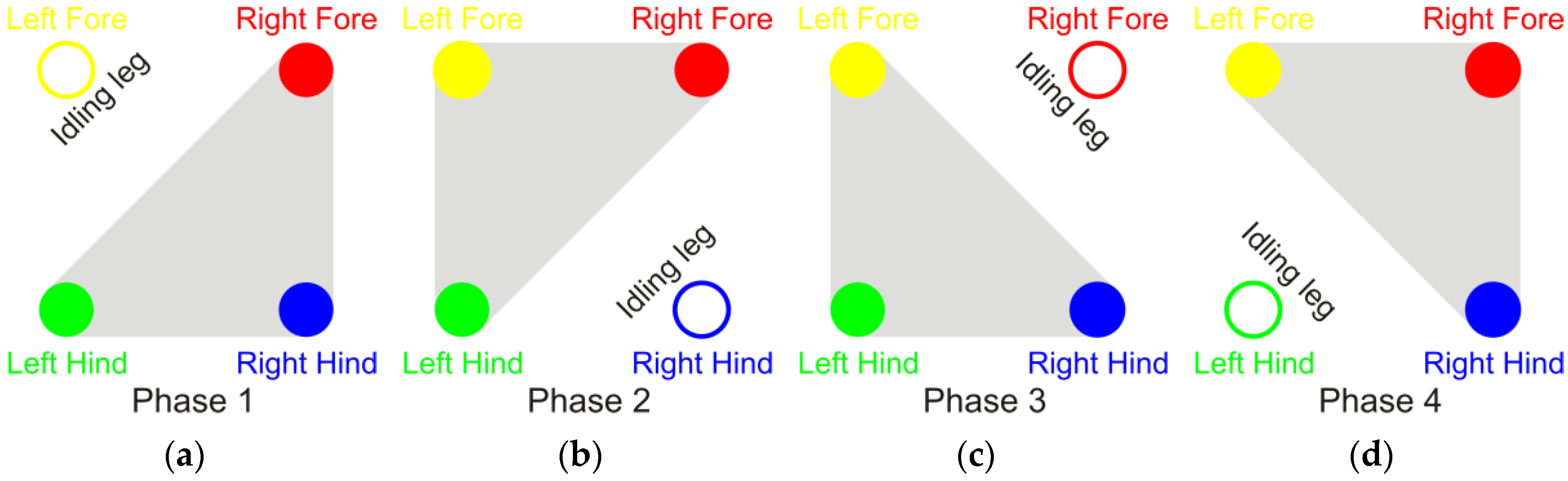Figure 10. An illustration of the static stability depending on the positional relationship between the center of gravity (COG) and the stability zone. (Left): Platform is stable; (Right): Platform is not stable.
Figure 10. An illustration of the static stability depending on the positional relationship between the center of gravity (COG) and the stability zone. (Left): Platform is stable; (Right): Platform is not stable.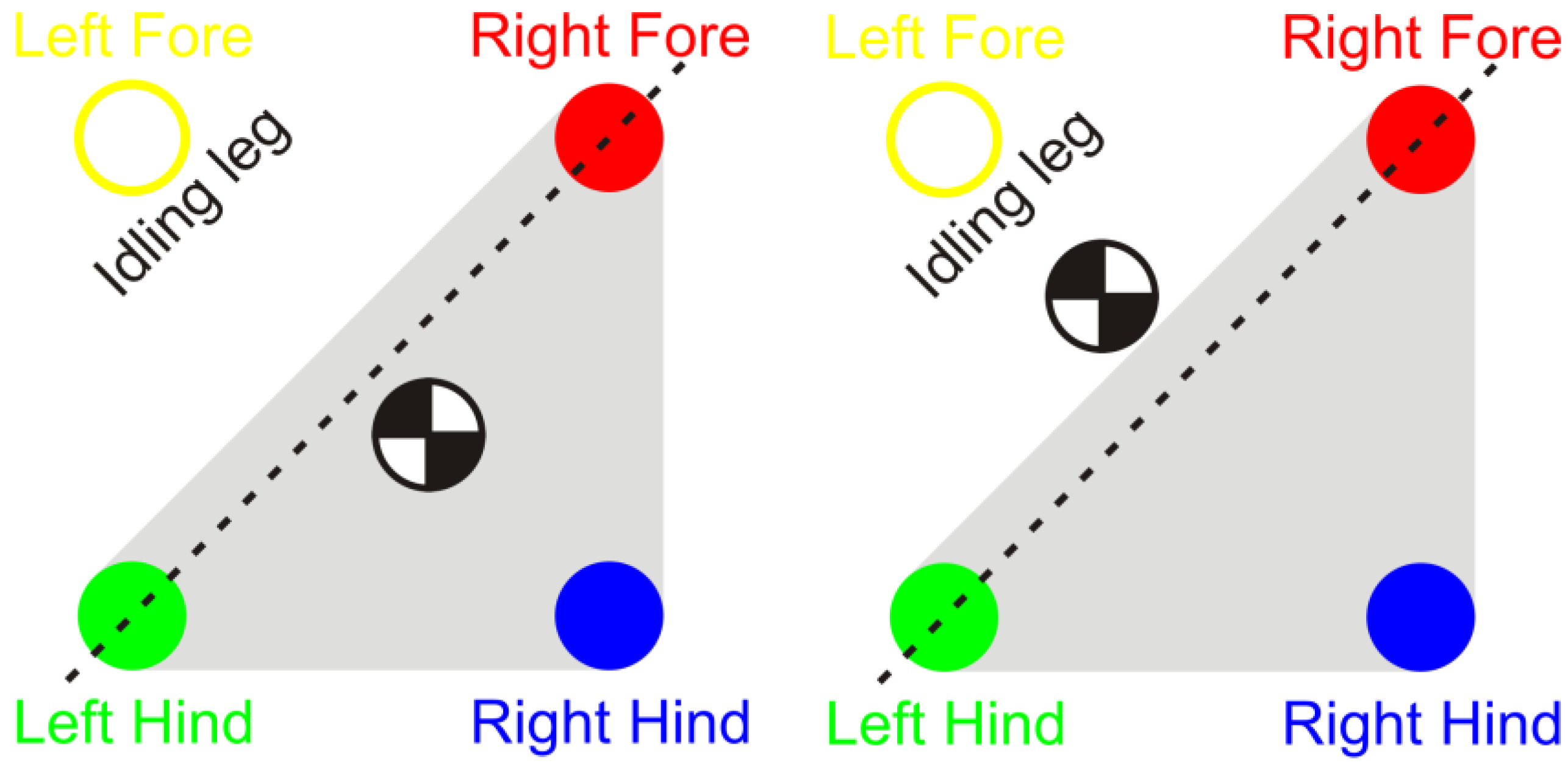Figure 11. Klann mechanism with definition of the links.
Figure 11. Klann mechanism with definition of the links.Figure 12. The static transformation from “Jam avoidance” to “Digging motion”. Top: Some steps of the transformation. Bottom: the generated input angles for the driving links for transformation. (a) time = 0.5 s; (b) time = 1.0 s; (c) time = 1.5 s; (d) time = 2 s; (e) generated input angles for Left-Fore, Left-Hind; (f) generated input angles for Right-Fore and Right-Hind.
Figure 12. The static transformation from “Jam avoidance” to “Digging motion”. Top: Some steps of the transformation. Bottom: the generated input angles for the driving links for transformation. (a) time = 0.5 s; (b) time = 1.0 s; (c) time = 1.5 s; (d) time = 2 s; (e) generated input angles for Left-Fore, Left-Hind; (f) generated input angles for Right-Fore and Right-Hind.Figure 13. The transformation from “Jam avoidance” to “Digging motion” by Transformation in Walking. Top: Some steps of the transformation. The transformation sequence is: Left-Fore—Right-Hind—Right-Fore—Left-Hind. Bottom-Left: (a) Transformation when t = 0; (b) Transformation when t = 1; (c) Transformation when t = 2; (d) Transformation when t = 3; (e) Transformation when t = 4; (f) Transformation when t = 5; (g) Transformation when t = 6; (h) Transformation when t = 7; (i) Transformation when t = 8; (j) The generated trajectories for the driving link; (k) The foot trajectory of leg “Right-Fore” during the transformation process.
Figure 13. The transformation from “Jam avoidance” to “Digging motion” by Transformation in Walking. Top: Some steps of the transformation. The transformation sequence is: Left-Fore—Right-Hind—Right-Fore—Left-Hind. Bottom-Left: (a) Transformation when t = 0; (b) Transformation when t = 1; (c) Transformation when t = 2; (d) Transformation when t = 3; (e) Transformation when t = 4; (f) Transformation when t = 5; (g) Transformation when t = 6; (h) Transformation when t = 7; (i) Transformation when t = 8; (j) The generated trajectories for the driving link; (k) The foot trajectory of leg “Right-Fore” during the transformation process.Figure 14. Zoomed area of the triangle frame of the Left-Fore leg.
Figure 14. Zoomed area of the triangle frame of the Left-Fore leg.Table 1. Identified novel foot trajectory patterns of interest for reconfiguration application.
 Default Curve Digitigrade Locomotion Jam AvoidanceThe standard foot directory of a Klann mechanism is similar to a kind of spider’s locomotion. The shape of this curve is quite similar to Digitigrade. Digitigrade walks on their digits or toes with short floor touch. A higher walking pattern and with a higher lift angle with a shorted stride than the standard one is appropriate for jam avoidance. Step Climbing Hammering Motion Digging MotionIn this case, the height of foot trajectory is about 500 (units), that is higher than normal trajectory. This curve provides a repeated short, rapid impact to an object with high force on a small area. This curve is of interest especially for exploration purposes.
Table 2. Initial input angle of four legs.
Table 2. Initial input angle of four legs.
Trajectory$θ A$ (rad)$θ B$ (rad)$θ C$ (rad)$θ D$ (rad)
Standard curve0.273.332.311.29
Jam avoidance0.643.442.511.58
Step climbing0.644.713.351.99
Digging1.743.082.632.18
Hammering2.583.843.423.0
Table 3. Walking phase synchronization for four legs (n: count of loop, starts from 0).
Table 3. Walking phase synchronization for four legs (n: count of loop, starts from 0).
StepLegPhase 1Phase 2Phase 3Phase 4
1Left Fore$θ A − 2 π n$$θ B − 2 π ( n + 1 )$$θ C − 2 π ( n + 1 )$$θ D − 2 π ( n + 1 )$
2Right Hind$θ D − 2 π n$$θ A − 2 π n$$θ B − 2 π ( n + 1 )$$θ C − 2 π ( n + 1 )$
3Right Fore$θ C − 2 π n$$θ D − 2 π n$$θ A − 2 π n$$θ B − 2 π ( n + 1 )$
4Left Hind$θ B − 2 π n$$θ C − 2 π n$$θ D − 2 π n$$θ A − 2 π n$
Table 4. Results of the static stability analysis of the synchronization.
Table 4. Results of the static stability analysis of the synchronization.
Phase 1Phase 2Phase 3Phase 4
Standard curve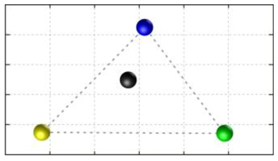Digitigrade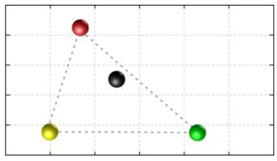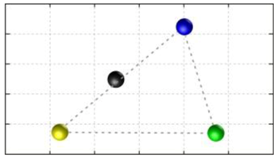Jam avoidanceStep climbing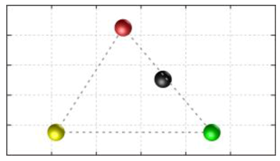Digging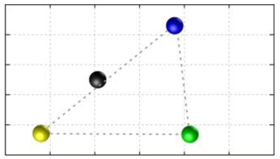HammeringTable 5. The initial and final state of the transfer leg in the transformation in walking.
Table 5. The initial and final state of the transfer leg in the transformation in walking.
VariablesBefore TransformationAfter Transformation
$θ$: input angle (rad)$θ A$$θ ′ B$
$θ ˙$: input velocity (rad/s)$η / T$$η ′ / T$
$l$: link dimension (mm)
$Δ y$: height offset (mm)0$P 8 y − P ′ 8 y$
Table 6. Results of the static stability analysis of the transformation ii -transformation in motion.
 Standard Curve Digitigrade Locomotion Jam Avoidance Step Climbing Digging Motion Hammering Motion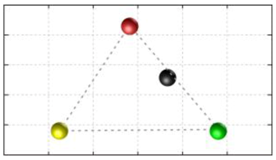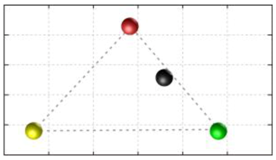Digitigrade Locomotion Standard Curve Jam Avoidance Step Climbing Digging Motion Hammering MotionJam Avoidance Standard Curve Digitigrade Locomotion* Step Climbing Digging Motion* Hammering MotionStep Climbing Standard Curve Digitigrade Locomotion * Jam Avoidance Digging Motion * Hammering Motion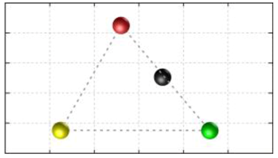Digging Motion Standard Curve Digitigrade Locomotion Jam Avoidance Step Climbing Hammering Motion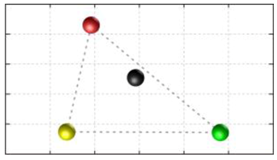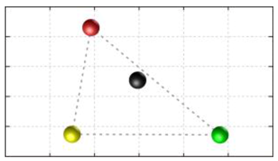Hammering Motion Standard Curve Digitigrade Locomotion * Jam Avoidance Step Climbing Digging Motion *